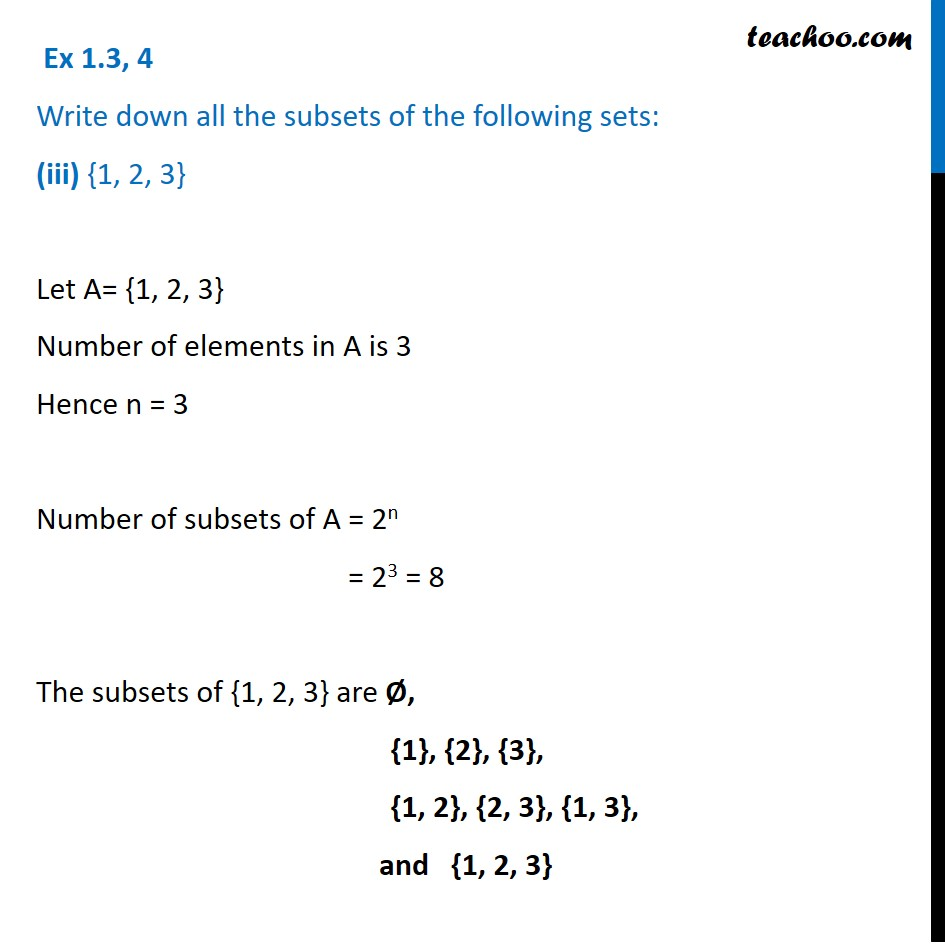1. Chapter 1 Class 11 Sets (Term 1)
2. Serial order wise
3. Ex 1.3

Transcript

Ex 1.3, 4 Write down all the subsets of the following sets: (iii) {1, 2, 3} Let A= {1, 2, 3} Number of elements in A is 3 Hence n = 3 Number of subsets of A = 2n = 23 = 8 The subsets of {1, 2, 3} are Ø, {1}, {2}, {3}, {1, 2}, {2, 3}, {1, 3}, and {1, 2, 3}